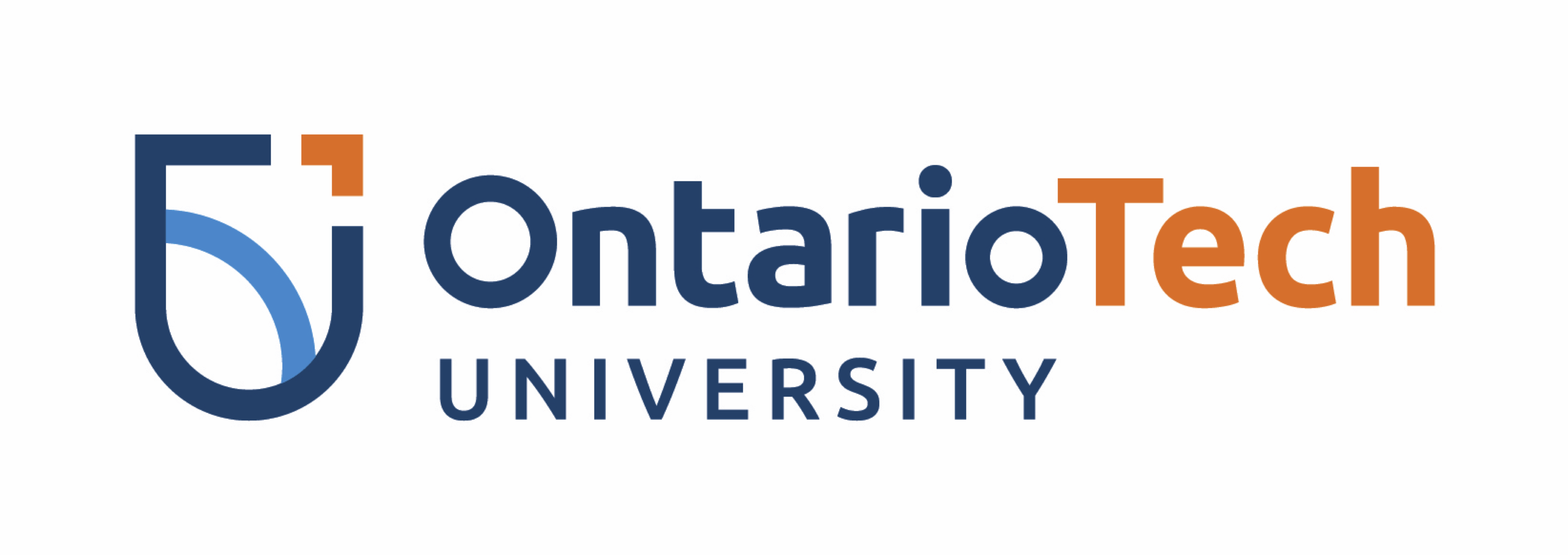# Machine Learning¶

Faisal Qureshi
http://www.vclab.ca

## Lesson Plan¶

• What is Machine Learning (ML)
• ML and deep learning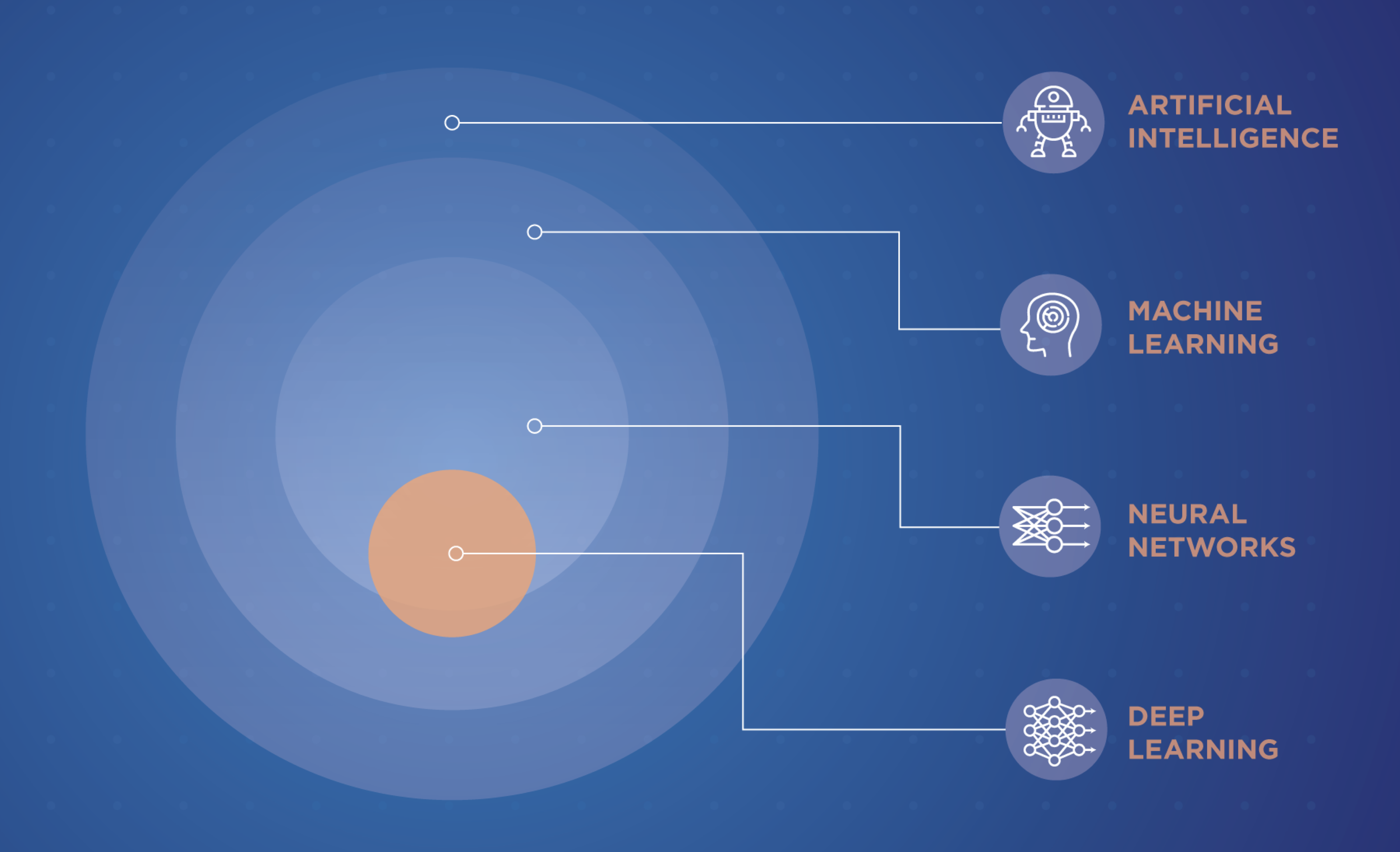Figure from the Scientist

## What Is Machine Learning?¶

• Mitchell, 1997:

A computer program is said to learn from experience $E$ with respect to some class of tasks $T$ and performance measure $P$ , if its performance at tasks in $T$ , as measured by $P$ , improves with experience $E$.

• "Subfield of artificial intelligence"
• More instructive: Machine learning is a means of building mathematical models of data.

## Supervised vs.Unsupervised Learning¶

### Supervised learning: Modeling relationships between measured data features and prescribed, associated targets (or labels)¶

• "Given input $A$, predict target $B$"
• Entails regression (continuous labels/targets) & classification (discrete targets/labels)
• Using established data measurements to infer targets/labels in as-of-yet unseen data
• Prediction accuracy can be evaulated from prescribed data

### Unsupervised learning: Models applied to data without any targets or labels¶

• "Letting the dataset speak for itself"
• Entails clustering, dimensionality reduction, and density estimation
• "What patterns can we infer from $A$? Which features of $A$ occur together frequently?"

## Supervised Learning¶

### Regression problems¶

• Inputs features $\mathbf{X}$ and generic (continuous) targets/labels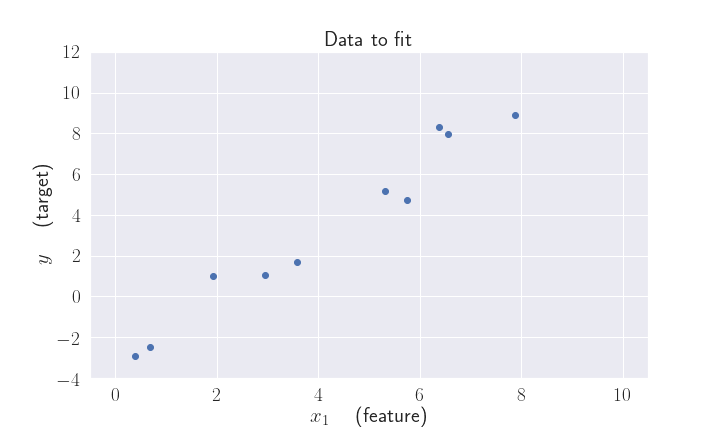Desired output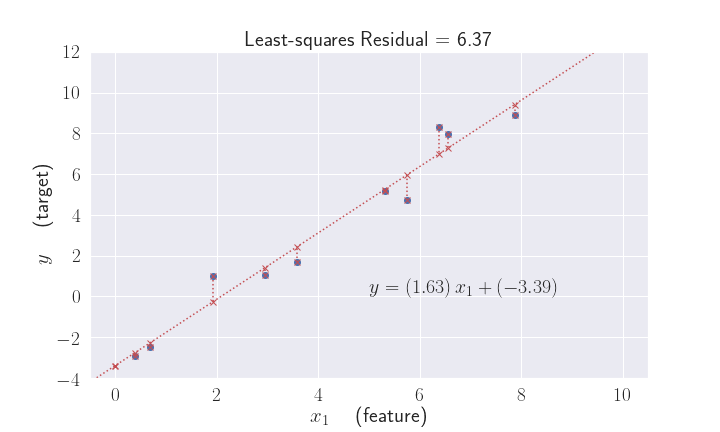### Classification problems¶

• Inputs features $\mathbf{X}$ and categorical/discrete targets/labels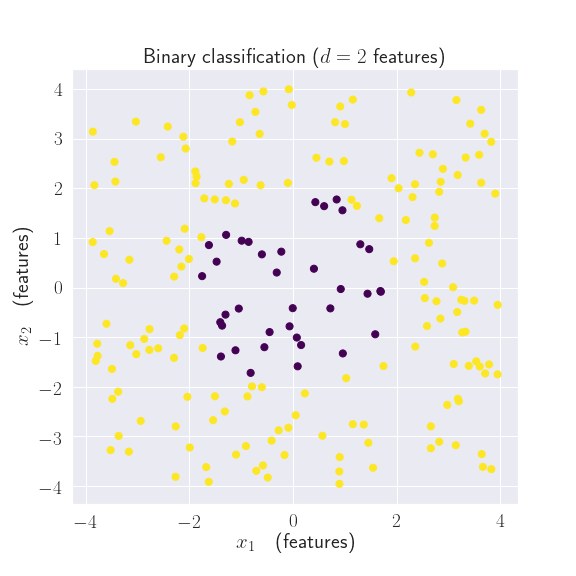Desired output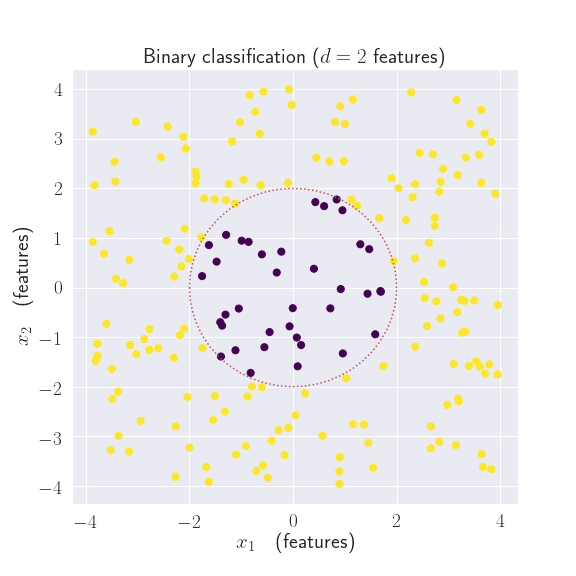## Unsupervised Learning¶

### Clustering problems¶

• No input/output pairs, just raw features $\mathbf{X}$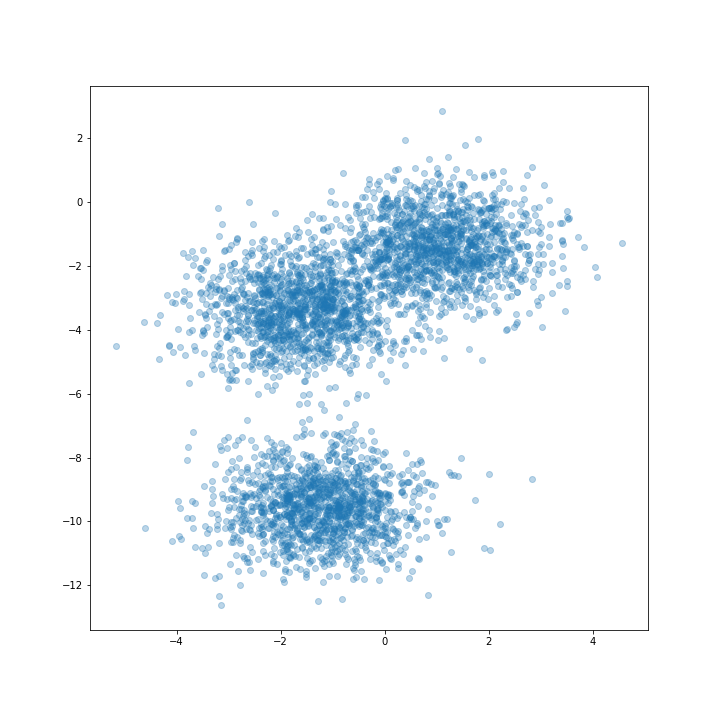Desired output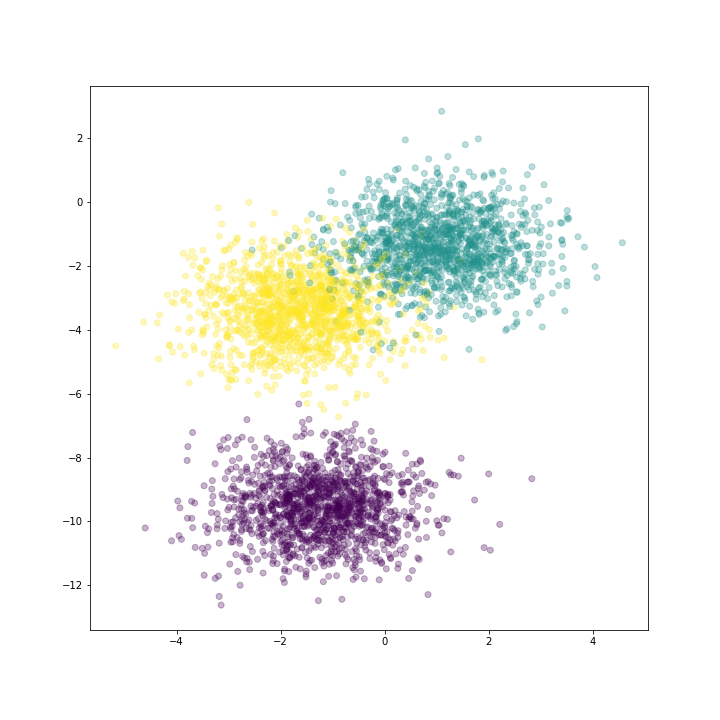### Other unsupervised learning problems¶

• Dimensionality reduction
• Fitting distributions
• Recommender systems
• Association analysis

## Machine learning --> deep learning¶

• model --> network, graphs
• parameters --> weights
• fitting --> learning
• test set performance --> generalization
• regression, classification --> supervised learning
• density estimation, dimensionality reduction, clustering --> unsupervised learning
• large grant ~50,000 dollars --> large grant ~1,000,000 dollars

## Afterward¶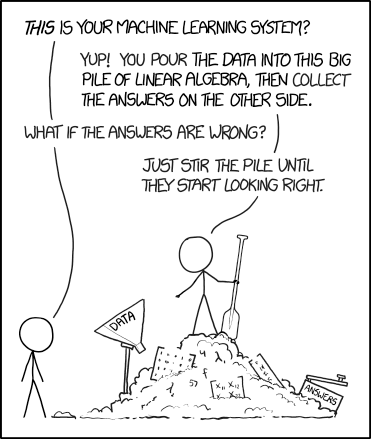Credit: https://xkcd.com/1838/In [ ]: